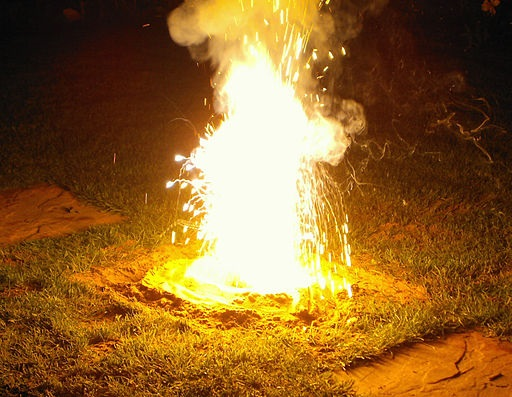10 minutes maximum! Can you do it in 5?

1. Which statement is true for ALL metals?
• A.   They are weak and brittle
• B.   They do not conduct electricity when solid
• C.   They melt at low temperatures when heated
• D.   Their atoms lose one or more electrons when they react
2. Lead is a typical metal. Which of the following is NOT a property of lead?
• A.   It can be hammered into shape
• B.   It has a low density
• C.   It forms covalent compounds
• D.   It conducts heat
3. Element Q is a solid element which forms a basic oxide, forms an alloy and is below hydrogen in the reactivity series. Element Q could be ...
• A.   copper
• B.   carbon
• C.   zinc
• D.   sulfur

4. Which one of the following is a metallic element?

 Electrical conductivity (solid) Electrical conductivity (liquid) A low low B low high C high low D high high

5. Which one of the following substances could be magnesium metal?

 Malleability Reaction with hydrochloric acid A bends no reaction B breaks no reaction C bends Reacts giving effervescence D breaks Reacts giving effervescence

6. Which of the following is true about the reactivity of metals?

 The more reactive a metal the ….. A more reactive metal will …… A greater its tendency to form positive ions not displace a less reactive metal from a compound B greater its tendency to form positive ions displace a less reactive metal from a compound C less its tendency to form positive ions not displace a less reactive metal from a compound D less its tendency to form positive ions displace a less reactive metal from a compound

7. Four metals reacted as follows:

 Metal With cold water With acid W Reacts very slowly Slow reaction X No reaction Slow reaction Y Fast reaction Violent reaction Z No reaction No reaction

The four metals, W, X, Y, Z in order of decreasing reactivity are ...
• A.   Y, W, X, Z
• B.   Y, W, Z, X
• C.   Z, X, W, Y
• D.   X, Z, W, Y
8. The results of two experiments are summarized in the following word equations:

Zinc + nickel oxide  zinc oxide + nickel

Nickel + platinum oxide  nickel oxide + platinum

These three metals in order of increasing reactivity are:

• A.   platinum, nickel, zinc,
• B.   nickel, platinum, zinc
• C.   zinc, nickel, platinum
• D.   nickel, zinc, platinum

9. Some reactions of three metals are listed in the table below:

 Metal Does metal react with dilute acid? Is the metal oxide reduced by carbon? R yes no S yes yes T no yes

The order of reactivity of these metals is:

 Most reactive to least reactive A R T S B R S T C S R T D T R S
10. A violent reaction occurs when iron(III)oxide reacts with aluminium powder. The equation for the reaction is:

Fe2O3 + 2Al  2Fe + Al2O3The substance that is oxidized in this reaction is ...

• A.   iron(III)oxide
• B.   aluminium
• C.   iron
• D.   aluminium oxide